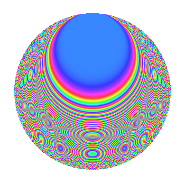# Properties

 Label 225.2.sLevel 225 Weight 2 Character orbit s Rep. character $$\chi_{225}(8,\cdot)$$ Character field $$\Q(\zeta_{20})$$ Dimension 80 Newform subspaces 1 Sturm bound 60 Trace bound 0

# Related objects

## Defining parameters

 Level: $$N$$ = $$225 = 3^{2} \cdot 5^{2}$$ Weight: $$k$$ = $$2$$ Character orbit: $$[\chi]$$ = 225.s (of order $$20$$ and degree $$8$$) Character conductor: $$\operatorname{cond}(\chi)$$ = $$75$$ Character field: $$\Q(\zeta_{20})$$ Newform subspaces: $$1$$ Sturm bound: $$60$$ Trace bound: $$0$$

## Dimensions

The following table gives the dimensions of various subspaces of $$M_{2}(225, [\chi])$$.

Total New Old
Modular forms 272 80 192
Cusp forms 208 80 128
Eisenstein series 64 0 64

## Trace form

 $$80q + 8q^{7} + O(q^{10})$$ $$80q + 8q^{7} + 8q^{10} - 4q^{13} + 20q^{16} - 40q^{19} - 88q^{22} - 16q^{25} - 128q^{28} - 20q^{34} - 4q^{37} + 12q^{40} + 32q^{43} - 4q^{52} - 8q^{55} - 12q^{58} + 160q^{64} + 64q^{67} + 136q^{70} + 76q^{73} + 80q^{79} + 84q^{82} + 52q^{85} - 56q^{88} - 80q^{94} - 236q^{97} + O(q^{100})$$

## Decomposition of $$S_{2}^{\mathrm{new}}(225, [\chi])$$ into newform subspaces

Label Dim. $$A$$ Field CM Traces $q$-expansion
$$a_2$$ $$a_3$$ $$a_5$$ $$a_7$$
225.2.s.a $$80$$ $$1.797$$ None $$0$$ $$0$$ $$0$$ $$8$$

## Decomposition of $$S_{2}^{\mathrm{old}}(225, [\chi])$$ into lower level spaces

$$S_{2}^{\mathrm{old}}(225, [\chi]) \cong$$ $$S_{2}^{\mathrm{new}}(75, [\chi])$$$$^{\oplus 2}$$

## Hecke Characteristic Polynomials

There are no characteristic polynomials of Hecke operators in the database